asycio实现TCP代理

asycio官方文档

ngrok原理简单分析

## 设计思路

### 内网穿透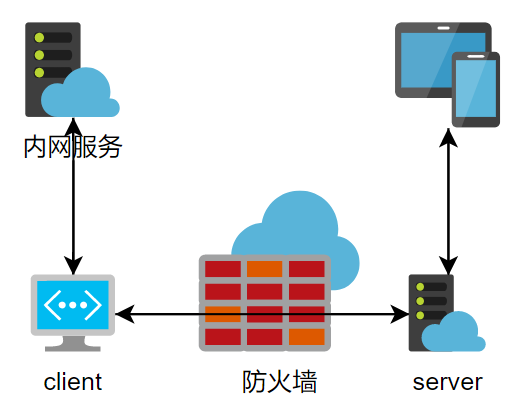### TCP代理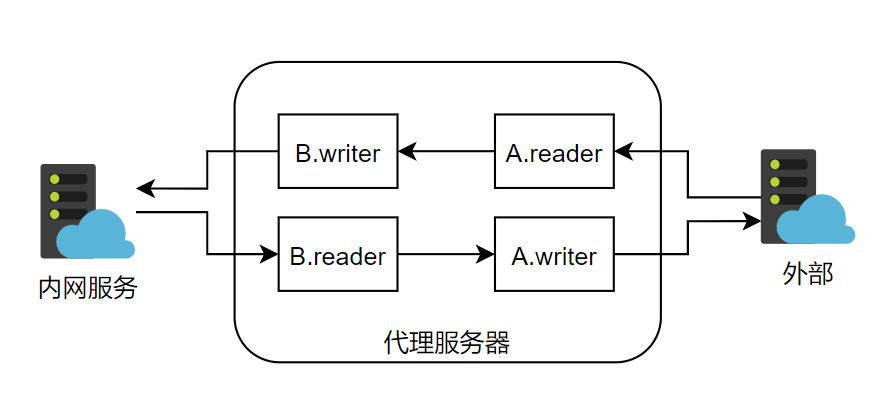async def join_pipe(reader, writer):
try:
finally:
writer.close()

try:
'jwzx.cqupt.edu.cn', 80
)
]
print(f'New TCP connection link { local_writer.get_extra_info("peername") !r} to { remote_writer.get_extra_info("peername") !r}')
finally:
local_writer.close()


### server与client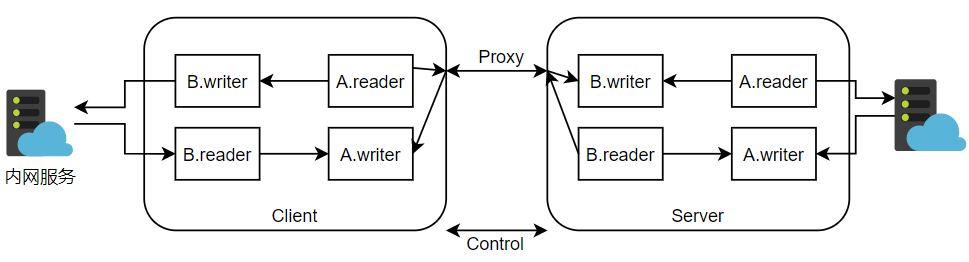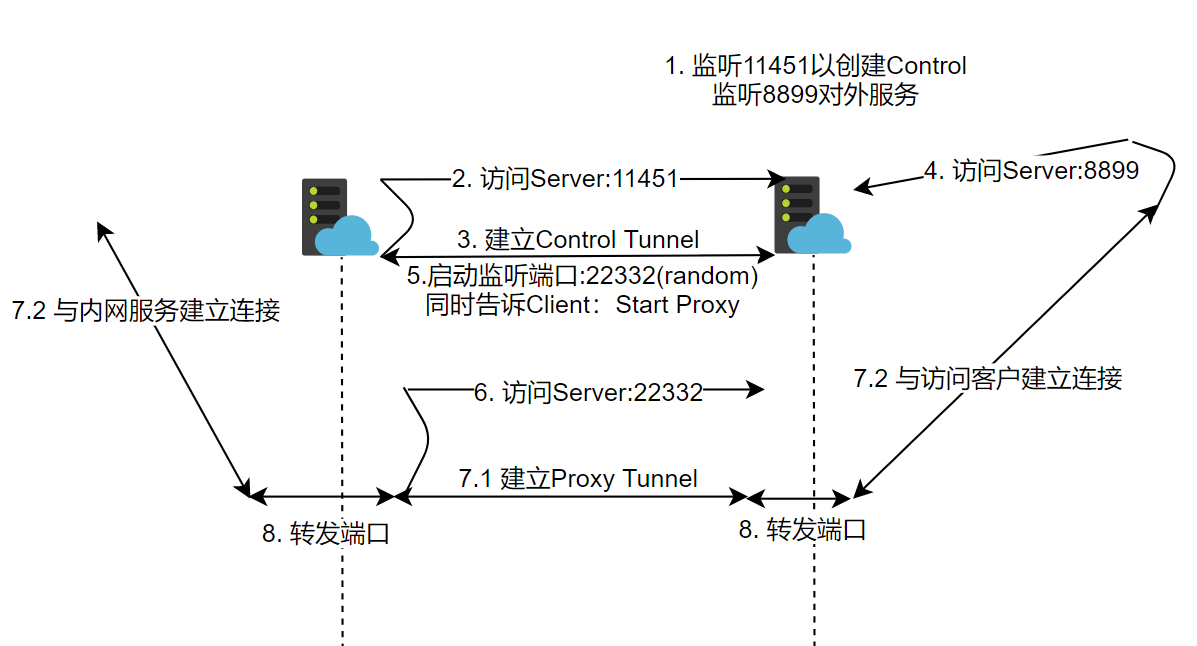Client在监听到Control发来的新请求以后会按照信息向新的端口发连接，成功建立Proxy，得到套接字A以后，再开始与内网服务器建立链接，得到套接字B，然后链接这两个套接字开始转发。

## 【树】2020ICPC小米网赛1G-Tree Projection

### 传送门

top：6 4 2 1 3 5

dfs：1 2 3 4 5 6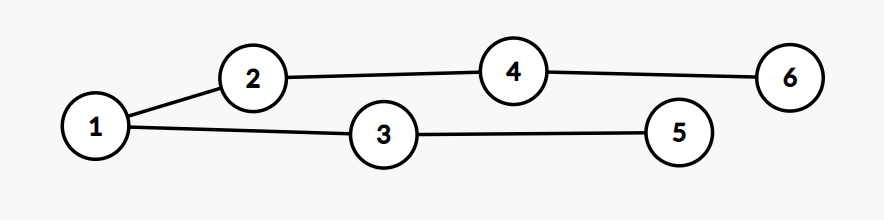#include<bits/stdc++.h>
using namespace std;
typedef long long LL;
typedef pair<int,int> pii;
mt19937 mrand(random_device{}());
int rnd(int x) { return mrand() % x;}
const int N = 2e5 + 233;
int a[N], b[N], pos[N];
int main()
{
// time_t startt = clock();

// cin.tie(0); cout.tie(0); ios::sync_with_stdio(0);
int n; 

## 【Splay】HDU20多校9-G Game

### 传送门

1 x y 是把第x行从下数第y个方块往左推一个。这会导致有一些方块悬空，这些悬空的方块会受到重力的影响下落。如果可以移动输出移动的方块数量。

2 x 是输出第x列的方块数量。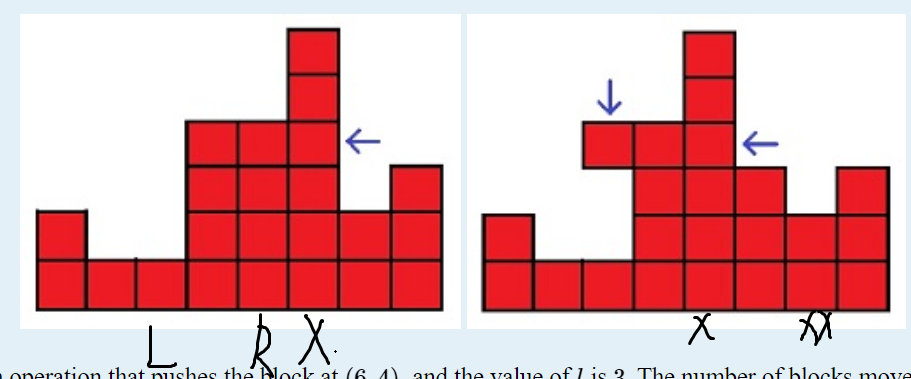1. X往左走一格，可以看作XX+1之间插入了一个(y-1)的数。
2. LX左边第一个小于y的数，假设L的是第rank个，那么R就是第rank+2个。LR之间那个数删掉即可。
3. L会加上前面那个柱子里掉下来的一些方块。

## 【状压DP】排列 – 阿里2020秋季校招

n < 1e15
m < 100

#include<bits/stdc++.h>
using namespace std;
typedef long long ll;
typedef pair<int,int> pii;
mt19937 mrand(random_device{}());
int rnd(int x) { return mrand() % x;}
const int N = 16, M = 110;
ll n, m;
ll f[1 << N][M];
vector<int> v;
bool vis;
int ze = 0;

int main()
{
// time_t startt = clock();

cin.tie(0); cout.tie(0); ios::sync_with_stdio(0);
int T;
T = 1;
while(T--)
{
cin >> n >> 

## 【贪心】CF448C – Painting Fence

### 传送门

#include<bits/stdc++.h>
using namespace std;
const int N = 5e3 + 233;
int a[N];
int solve(int l, int r, int u)
{
if(l > r) return 0;
int h = a[l], idx = l;
for(int i = l; i <= r; i++) h = a[i] < h ? a[idx = i] : h;
return min(r - l + 1, solve(l, idx - 1, h) + h - u + solve(idx + 1, r, h));
}
int 

## 【插头DP】Yajilin – 20HDU多校9Y

### 传送门

#pragma GCC optimize(3)
#include<bits/stdc++.h>
using namespace std;
typedef long long ll;
typedef pair<int,int> pii;
mt19937 mrand(random_device{}());
int rnd(int x) { return mrand() % x;}
const int N = 100007;
int n;
int a, state[N], dp[N], now;
int G(int k, int x) {return (k >> (x << 1)) & 3;} // 轮廓线k的第x位状态
int F(int k, int x) {return k << (x << 1);} // 编码第x位的状态k
// unordered_map<int, int> st;
int fst[N], pre[N], mk[N], mt, tot;
void init()


## 【KMP|DP】不含子串的最少删除次数

#include<bits/stdc++.h>
using namespace std;
typedef long long ll;
typedef pair<int,int> pii;
mt19937 mrand(random_device{}());
int rnd(int x) { return mrand() % x;}
int ne, f;
char str;
char p = " 0010";
int T;
int main()
{
// time_t startt = clock();
int m = 4;
for(int i = 2, j = 0; i <= m; i++)
{
while(j && p[i] != p[j 

## 【二分图匹配】Go Running – HDU20多校4G

### 传送门

#include<bits/stdc++.h>
using namespace std;
typedef long long ll;
typedef pair<int,int> pii;
mt19937 mrand(random_device{}());
int rnd(int x) { return mrand() % x;}
const int N = 1e5 + 233;
unordered_map<int, int> ux, uy;
int nx[N], 

## 【kmp|线性基】Kidnapper’s Matching Problem-HDU20多校8K

### 传送门

1. a_ib_i均能用这个线性基表示出来，那么异或出来的数一定在群里。
2. a_ib_i只有一个不能被线性基表示出来，那么异或出来的数肯定不在群里。

3. 若两个数都不能被线性基表示出来，异或结果是可能在群里的。当且仅当把能用S表示出来的部分删掉后a’=b’。这个其实蛮明显的，比赛的时候没想到。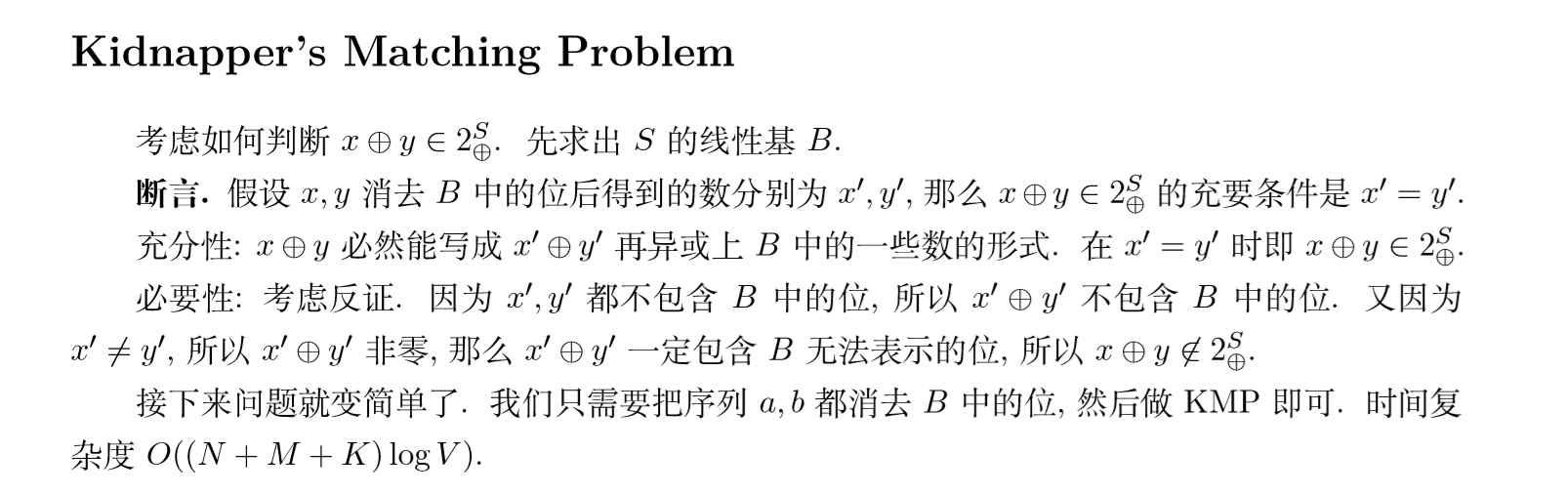#include<bits/stdc++.h>
using namespace std;
typedef long long ll;
typedef pair<int,int> pii;
mt19937 mrand(random_device{}());
int rnd(int x) { return mrand() % x;}
int n, m, k;
const int mod = 1e9 + 7;
const 

## 【异或最小生成树】Graph – 20牛客多校5-B

### 传送门

#include<bits/stdc++.h>
using namespace std;
using namespace std;

const int N = 100010, M = 3000000;

int a[N], son[M], idx;

void insert(int x)
{
int p = 0;
for (int i = 30; i >= 0; i -- )
{
int &s = son[p][(x >> i) & 1];
if (!s) s = ++ idx;
p = s;
}
}

int search(int x)
{
int p = 0, res = 0;